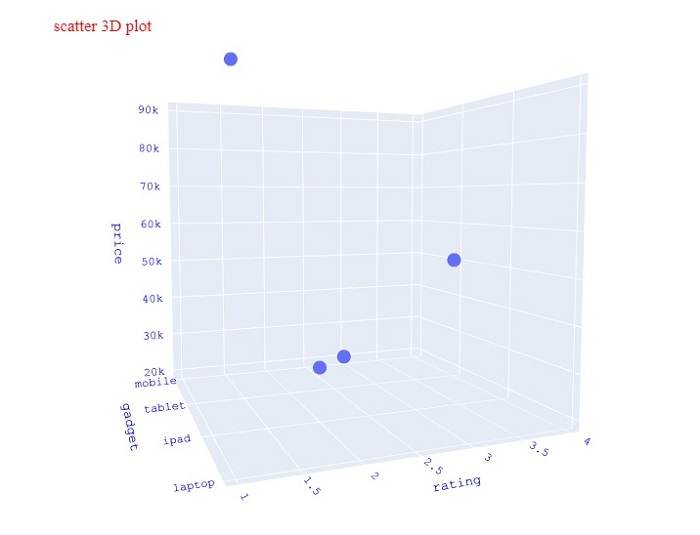# How to show legend and label axes in 3D scatter plots in Python Plotly?

Plotly is an open source Python library for creating charts. Python users can use Plotly to create interactive web-based visualizations that can be displayed in Jupyter notebooks, saved to standalone HTML files, or served as a part of web applications using Dash.

In this tutorial, we will show how you can use Plotly to display legend and label axes in a 3D scatter plot.

• Here, we will use plotly.express to generate figures. It contains a lot of methods to customize the charts and render them into HTML format.

• We will use the Pandas module can be used to create generate DataFrame.

• In addition, we will use the plotly.graphs_obj() method to generate the graph with different plots.

Follow the steps given below to show the legend and label axes.

Step 1

Import the plotly.express module and alias as px.

import plotly.express as px


Step 2

Create a dataframe using Pandas.

data = {
'rating' :[3,4,2,1],
'price':[20000,50000,30000,90000]
}
df = pd.DataFrame(data)


Step 3

Use the scatter_3d() method to create a Scatter 3D plot by applying the X and y coordinate values −

# Create scatter_3d plot
fig = px.scatter_3d(
y = 'rating',z = 'price',
title="scatter 3D plot"
)


Step 4

Use the update_layout() method to update the layout with the following properties −

fig.update_layout(
font_family="Courier New",
font_color="blue",
title_font_family="Times New Roman",
title_font_color="red",
legend_title_font_color="green"
)


## Example

The complete code to display legend and label axes in a 3D scatter plot is as follows −

import plotly.express as px
import pandas as pd
# Create DataFrame
data = {
'rating' :[3,4,2,1],
'price':[20000,50000,30000,90000]
}

df = pd.DataFrame(data)

# Create scatter_3d plot
fig = px.scatter_3d(df, x = 'gadget', y = 'rating',z = 'price', title="scatter 3D plot", width=716, height=750)

# Update the figure layout
fig.update_layout(
font_family="Courier New",
font_color="blue",
title_font_family="Times New Roman",
title_font_color="red",
legend_title_font_color="green"
)
fig.show()


## Output

On execution, it will show the following output on the browser −